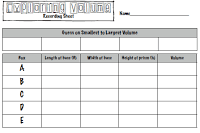Select Page

# Let’s Explore Volume

Mar 3, 2016

When you and I learned how to explore volume in math we memorized length x width x height and we knew each time if we computed correctly we would get the correct answer.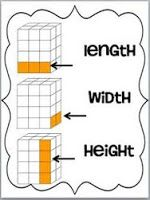So much of what we do with our schools at Strategic Intervention Solutions is the application of the 8 Mathematical Practice Standards. Our teachers discover that we as “teachers” are not the giver of all information and the more we can use inquiry-based hands on learning with our 21st century students, the more they will see the application of the math skills taught.

We want to take you into a 5th grade classroom where students are doing what we like to call a “Math Exploration Task Lab” about mid-way through their unit of study on the exploration of volume. We like to do “Math Exploration Task Labs” at the beginning of the unit and if possible at the end in order to gain how much knowledge the students have on the content being taught.

In this classroom there were 4 distinct stations set up where students worked collaboratively with their group to solve the task at hand.
Station #1: Exploring Volume
Station #2: Dixie Cup Fill
Station #3: Centimeter Cube Box
Station #4: Scavenger Hunt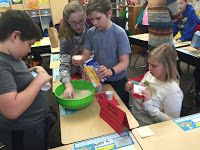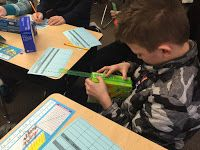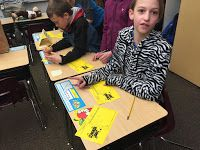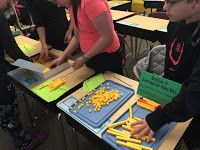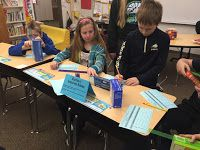Print these tent cards on colored card stock and set them out at each station with the materials needed.  The activity tents explain what students need and the directions for the stations.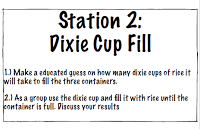Print these recording sheets for students to record their work for each station.